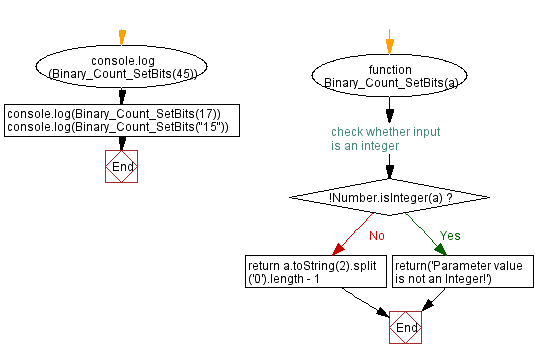# JavaScript - Number of 0 bits in a binary representation

## JavaScript Bit Manipulation: Exercise-3 with Solution

Write a JavaScript program to count 0 bits in the binary representation of a given integer.

Test Data:
(45) -> 2
(17) -> 3
(15) -> "Parameter value is not an Integer!"

Sample Solution:

JavaScript Code:

``````function Binary_Count_SetBits(a)
{
// check whether input is an integer
if (!Number.isInteger(a))
{
return('Parameter value is not an Integer!')
}
return a.toString(2).split('0').length - 1
}
console.log(Binary_Count_SetBits(45))
console.log(Binary_Count_SetBits(17))
console.log(Binary_Count_SetBits("15"))
```
```

Sample Output:

```2
3
Parameter value is not an Integer!
```

Flowchart:Live Demo:

See the Pen javascript-bit-manipulation-exercise-2 by w3resource (@w3resource) on CodePen.

* To run the code mouse over on Result panel and click on 'RERUN' button.*

Improve this sample solution and post your code through Disqus

What is the difficulty level of this exercise?

Test your Programming skills with w3resource's quiz.

﻿